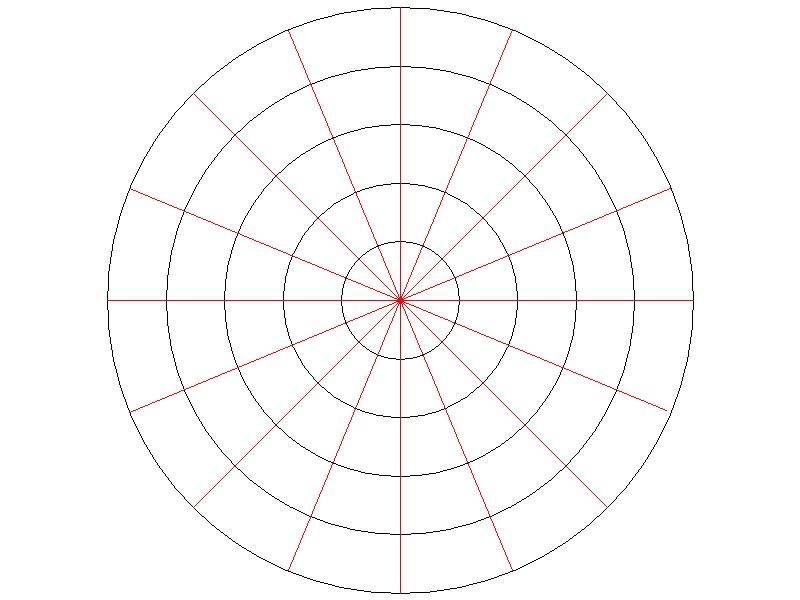# Force needed turn robot wheel in stationary position

bluedragon
How much force is required to turn the front wheel of a 4 wheel robot that weighs 200lb. Assume the wheel is 6inches wide, has a diameter of 12 inches (rubber). I would like to know how much force is required to turn a single wheel left or right when it is in stationary (non-moving) position.

If anyone can help, can you please break down the math? I would like to be able to scale this down (or up) to explore various weight sizes.

jackwhirl
You mean to turn the wheels as you would by turning the steering wheel of a stopped car, right?

bluedragon
@jackwhirl yeah similarly, but each wheel is independent. and there is no steering column or pinion. The wheels are attached to the frame and pivot on an axis at the center of the wheel

Gold Member
The detail problem is almost unsolvable but you can get a working answer reasonably easily .

There is a contact zone between the bottom of the tyre and the road . Make the approximation that this zone is circular and that the steering axis goes through the centre . Make the further approximation that the wheel load is uniformly distributed over the contact zone .

Divide the contact zone into several nested annular sub zones . Work out the friction torque for each sub zone and sum to get the total friction torque .

It is easiest to use a relatively small number of sub zones and get the answer numerically but you can use calculus if preferred ..

Thus is essentially the same procedure as used to work out the load carrying capacity of clutch plates .

•jackwhirl
jackwhirl
Divide the contact zone into several nested annular sub zones .
I'm with you until this point, Nidum. Why annular, instead of rectangular or even a line?

Gold Member
It comes from dividing the contact zone into many segments using concentric circles and equally spaced radial lines .The torque contribution from each segment is then very easy to calculate . It turns out though that the number of segments per annular ring cancels in the calculations and that the basic annular subzone dimensions are all that is needed .

Circular sub division is easiest because of the assumed circular shape of the contact zone ..

Last edited:
Gold Memberjackwhirl
Circular sub division is easiest because of the assumed circular shape of the contact zone ..
Which would obviously be the case if the wheel is spherical, or on its side. I just don't see where you find that in the original post.

Gold Member
With the sparse data available there is no way of determining the exact shape of the contact zone or the distribution of load over the contact zone .

Assuming a circular contact zone and uniform load distribution does allow an approximate value for the steering torque to be obtained .

There are more complex versions of the basic calculation using rectangular or elliptic contact zones and load distribution based on an empirical rule . There are also more comprehensive analytic solutions and linear and non linear FEA .

For the present purpose though the circular contact zone model is the best that can be done . Calculate a value . Multiply by a safety factor for design purposes .